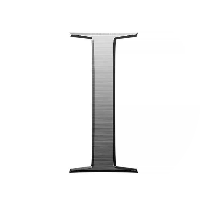# The Foundation: Logics and Proofs

This quiz contains multiple-choice problems on propositions, logic operations and circuits, implications, De Morgan’s law, statements types, tautologies, logical equivalences, quantifiers, inference and proofs types.

Start Quiz

What is the value of x after this statement, assuming the initial value of x is 5? ‘If x equals to one then x=x+2 else x=0’.

1 3 0 2

Which of the following statements is a proposition?

Get me a glass of milkshake.

God bless you!

What is the time now?

The only odd prime number is 2.

What is the truth value of “4 + 3 = 7 or 5 is not prime”?

False

True

What is the truth value of ‘9 is prime then 3 is even’?

False

True

P: “If Sahil bowls, Saurabh hits a century.”

Q: “If Raju bowls, Sahil gets out on the first ball.”

If P is true and Q is false, which of the following statements is true?

“Raju bowled and Sahil got out on first ball.”

“Raju did not bowl.”

“Sahil bowled and Saurabh hits a century.”

“Sahil bowled and Saurabh got out.”

Which of the following statements is true?

If the Sun is a planet, elephants will fly

3 + 2 = 8 if 5 - 2 = 7

1 > 3 and 3 is a positive integer

-2 > 3 or 3 is a negative integer

P: “We should be honest.”

Q: “We should be dedicated.”

R: “We should be overconfident.”

Which logical expression best represents the statement, “We should be honest or dedicated but not overconfident”?

~P V ~Q V R

P ∧ ~Q ∧ R

P V Q ∧ R

P V Q ∧ ~R

P: “This is a great website.”

Q: “You should not come back here.”

Which logical expression best represents the statement, “This is a great website, and you should come back here”?

~P V ~Q

P ∧ ~Q

P V Q

P ∧ Q

P: “I am in Delhi.”

Q: “Delhi is clean.”

Which of the following statements corresponds to q ^ p (q and p)?

“Delhi is clean and I am in Delhi.”

“Delhi is not clean or I am in Delhi.”

“I am in Delhi and Delhi is not clean.”

“Delhi is clean but I am in Mumbai.”

P: “I am in Bangalore.”

Q: “I love cricket.”

Which of the following statements corresponds to q -> p (q implies p)?

“If I love cricket, then I am in Bangalore.”

‘If I am in Bangalore, then I love cricket.”

“I am not in Bangalore.”

“I love cricket.”

Which of the following bits is the negation of the bits 010110?

111001

001001

101001

111111

Which of the following option is suitable, if A is 10110110, B is 11100000, and C is 10100000?

C = A or B

C = ~A

C = ~B

C = A and B

How many bit strings of length 4 are possible such that they contain 2 ones and 2 zeroes?

4

2

5

6

If a bit string of length 5 containing {0, 1} has no more than 2 ones in it, then just how many such bit strings are possible?

14

12

15

16

If A is 001100 and B is 010101, then what is the value of A (Ex-or) B?

000000

111111

001101

011001

Quiz/Test Summary
Title: The Foundation: Logics and Proofs
Questions: 15
Contributed by: